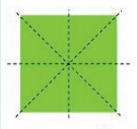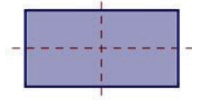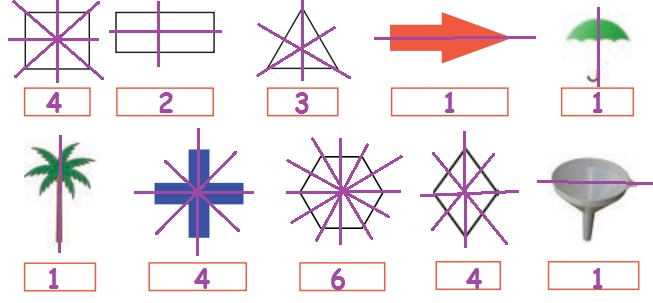Home | | Maths 5th Std | Symmetry in familiar 3-D shapes

# Symmetry in familiar 3-D shapes

Able to explore symmetry in familiar 3-D shapes like in alphabets intuitively

Able to explore symmetry in familiar 3-D shapes like in alphabets intuitively.

For example, if we cut an apple into two equal halves, we observe that two parts are in symmetry.Symmetry is an important geometrical concept commonly seen in nature and is used in every field of our life. Artists, manufactures, designers, architects and others make use of the idea of symmetry.

Symmetry refers to the exact match in shape and size between two halves of an object.

When we fold a picture into two halves, and if both the halves match exactly then we say that the picture is symmetrical.Line of symmetry:

When a line divides a given figure into two equal halves and it matches exactly, then we say that the figure is symmetrical about the line. This line is called the line of symmetry or axis of symmetry.Example4 lines of symmetry: We can divide a square in 4 ways symmetrically.2 lines of symmetry: We can divide a rectangle in 2 ways symmetrically.

Project:

1. List out 2 symmetrical objects that you know.

2. Tick the picture which is symmetrical3. Complete the other half to make the given figure symmetric.4. Draw the lines of symmetry for the following figures.Think it

1. Can we divide the irregular solids, symmetrically? If no, why?

Answer: No, because when we draw a line and divide the object into equal halves, both the sides will not match with one another. It is not symmetrical.

2. Write the English alphabets that can't be divided symmetrically?

Answer: F G J L N P Q R S T Y Z

3. Write the alphabets of English which are symmetrical. Further find whther the symmetry is horizontal and vertical.

Answer: A B C D E H I K M O U V W X

HIOX have horizontal and vertical symmetry.

AMUV - Vertical symmetry

BCDEK - Horizontal symmetry

4. Circle has many lines of symmetry. Is it true? why?

Answer: True. We can draw many lines of symmetry for a circle. Each line will give a symmetry for the circle.

5. Find the three numbers between 1 and 9 that can be divided symmetrically.

6. Find two numbers between 1 and 9 with two lines of symmetry.

Project

Write down 26 English alphabets in a chart. Draw the lines of symmetry for the letters. Circle the remaining letters that are not symmetrical.Do you know?

Tajmahal in Agra is a symmetrical monument.Tags : Geometry | Term 1 Chapter 1 | 5th Maths , 5th Maths : Term 1 Unit 1 : Geometry
Study Material, Lecturing Notes, Assignment, Reference, Wiki description explanation, brief detail
5th Maths : Term 1 Unit 1 : Geometry : Symmetry in familiar 3-D shapes | Geometry | Term 1 Chapter 1 | 5th Maths